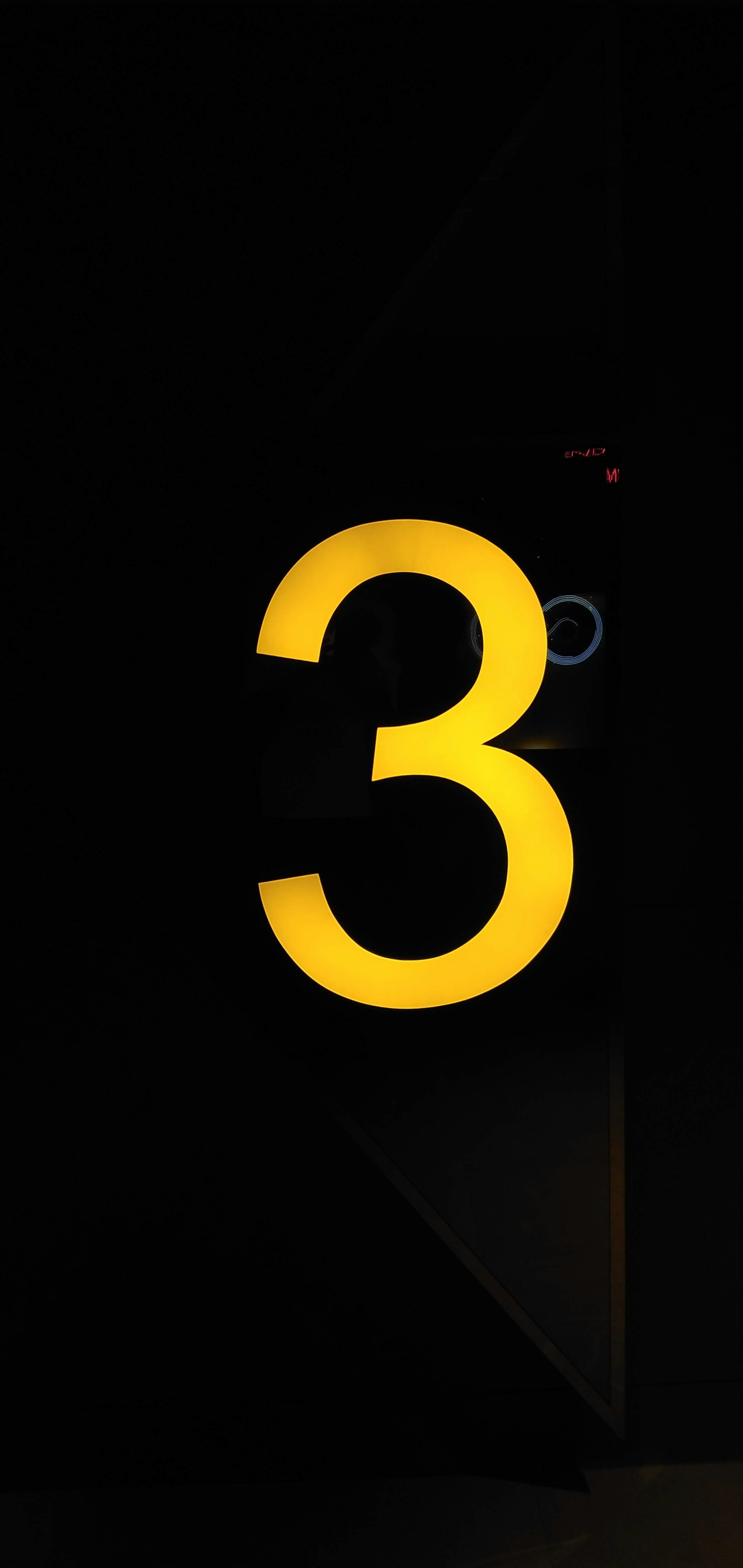Arsalan Wasim

# Max PoolingConvolutional layers are excellent for recording the precise location of features in the input image. A big problem of convolution neural networks is that they calculate the specific features from a given image and any change in the image such as zooming, panning, or any other physical change results in incorrect predictions. In other words, you can say that they are translation variant, meaning that they will be changed if there is any physical translation.

To make the learned features more robust, max pooling is commonly used. Max pooling results in downsampling the input image by learning the dominant feature located in the result of the previous convolution. Let us clarify this with an example.

Suppose that we have an input two-dimensional padded matrix:We will use a vertical line detector kernel to detect vertical lines. The filter is also a two-dimensional matrix and would look as follows:The result of convolution operation would look as:Now we will use the max-pooling layer to learn the most dominant feature from the resultant matrix, the operation looks as follows:

max(0.0 ,2.0)           =     [3.0]

(0.0, 3.0)

Since our output from the convolution operation is a (3,3) matrix, max-pooling would only look at the first (2,2) window and by default max pool layer has a stride of 2. So it will skip the others as it will be out of its scope but would output the most dominant feature which is [3.0].

Max pooling makes the convolution operation faster as it is very easy to compute. It also makes the learned features translation-invariant. In other words, if the input image is suffering from zooming, panning, brightening, etc, then it would still calculate the best result most of the time.